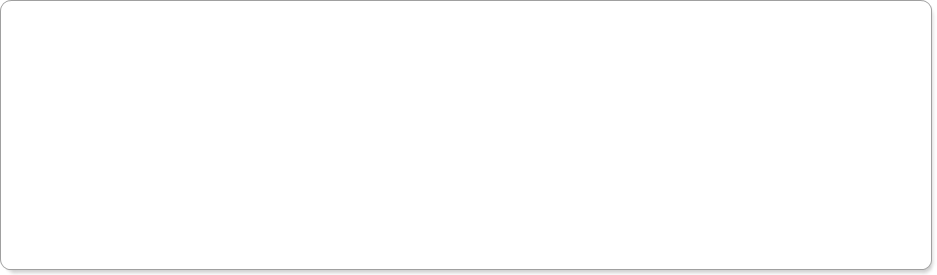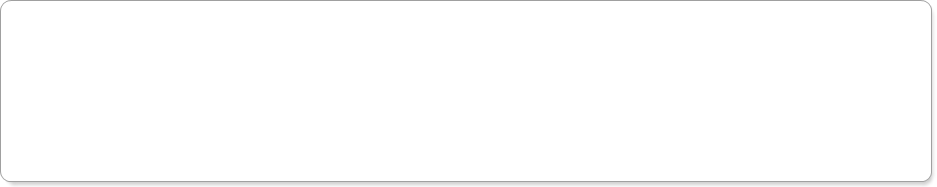Robotics 1
DC Motors Torque and Speed
QuizYour ID Code:
Question 2:  Which of these is true?
Question 3:  Suppose you test a motor at 12V and observe that the motor turns at 500 rpm.  What is the motor velocity constant?  Round your answer to 3 places after the decimal.  Also, enter only a number, don't enter units.
Question 4:  Suppose you know that the motor in Question 3 will spin at 500 rpm at 12V.  Now, suppose that you turn the 12V signal on 1/5 of the time, and off 4/5 of the time.  How fast will the motor spin?  Give your answer in units of rpm, but don't enter units; just enter a number.
Question 5:  Suppose you have a clock speed of 12MHz and you set the period of the PWM to 400 'ticks'.  How much time, in milliseconds, is the period?  Round your answer to 5 places after the decimal.
Question 6:  Suppose I set the period of the PWM to 100 'ticks'.  If I know that the motor spins at 500rpm when the compare value is 100, how fast should I expect the motor to spin at a compare value of 30?  Round your answer to 3 places after the decimal.
Question 7:  What is meant by a motor's 'no-load speed' or 'free-run speed'?
Question 8:  What is meant by a motor's 'stall torque'?
Question 9: Suppose you have a motor with a no-load speed of 290 rpm and a stall torque of 0.233 Nm.  You can assume that the torque/speed curve is a straight line between these points.  What is the maximum speed at which the motor can exert 0.1 Nm of torque?  Round your answer to 3 places after the decimal.
Question 10:  For Question 9, suppose you have attached the shaft of this motor to a pulley with a radius of 1cm.  What is the maximum mass, in kg, that this motor can lift at a speed of 60 rpm?  Round your answer to 3 places after the decimal.
Undergraduate/Graduate Questions (both undergraduates AND graduates should answer these questions):
Question 11:  Suppose you have a joint attached to a link as shown here.  The link length is 0.2m and the mass of the link is 0.3kg.  You want to be able to complete a 180-degree move in 0.2 seconds.  What is the maximum velocity at which the motor has to move, in units of radians per second?  Round your answer to three places after the decimal.
Question 12:  For Question 11, what is the acceleration, in units of radians/second^2, that is required?  Round your answer to three places after the decimal.
Question 13:  For Questions 11 and 12, what is the torque, in units of Nm, required for the acceleration?  Round your answer to three places after the decimal.
Question 14:  For Questions 11-13, now suppose that we have rotated the joint 90 degrees so that the link moves in a direction parallel to gravity.  How much additional torque, in units of Nm, is required to counter the force of gravity on the link?  Round your answer to three places after the decimal.
Question 15:  Now suppose that you have added a payload of 0.2kg.  How much additional torque, in units of Nm, is required to move this payload?  Include both the torque required to accelerate the mass and the torque required to counter the force of gravity on the mass.  Round your answer to three places after the decimal.
Question 1: What does the term 'servo' generally refer to?
A 'servo' is a device that has both a motor and a sensor in one device.
A 'servo' is any device that is used for producing rotational motion.
A 'servo' is any device for producing motion.
A 'servo' is a device for controlling motion in remote-control devices.
The higher the current, the higher the motor speed
The higher the voltage, the lower the motor speed
The higher the voltage, the higher the motor speed
The higher the current, the lower the motor speed
The speed the motor turns when the current is maximum
The speed the motor turns when the torque is maximum
The speed the motor turns when the current is minimum
The speed the motor turns when the torque is minimum
The amount of torque when the speed is maximum
The amount of torque when the speed is minimum
The amount of torque when the voltage is maximum
The amount of torque when the voltage is minimum
Graduate Questions (ONLY graduates need to answer these questions):